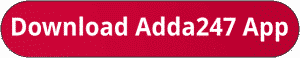Latest Banking jobs   »   RBI Grade B Phase 1 quant

# Quantitative Aptitude Quiz For RBI Grade B Phase 1 2023 – 07th July

Q1. Abhi invested some amount on scheme ‘P’ which offer CI at the rate of 15% p.a.. After 2 years he got Rs. 1032 as interest. Abhi invest the amount he got from scheme ‘P’ in scheme ‘Q’ which offer 15% p.a. at SI for 4 years. Find the total interest he earned from scheme P and scheme Q together?
(a) 3571.2
(b) 3715.2
(c) 4232
(d) 3148
(e) 3379.2
Q2. S₁ is a series of five consecutive multiple of three, whose sum is 180 and S₂ is the series of four consecutive multiple of four whose second smallest number is 13 more than second highest number of S₁ series. Find the average of smallest number of S₁ series and highest number of S₂ series.
(a) 51
(b) 49
(c) 47
(d) 45
(e) 43Q4. A milkman mixed 32 litre of water in 48 litre of milk and sold 30 litre of mixture. Then he again added some milk and water whose quantity is in the ratio 5 : 4 in the remaining mixture so that ratio of water and milk become 7 : 10. Now he sell 25% of mixture, then find the amount of milk in the rest of the mixture.
(a) 30 liter
(b) 21 liter
(c) 15 liter
(d) 22.5 liter
(e) 24 liter
Q5. A man swimming at 75 percent of his usual speed can covers 128 km in 8 hours in downstream. In the return journey he swims at 50% of his usual speed and take 32 hours. Speed of man is what percent more than speed of stream?
(a) 100%
(b) 200%
(c) 300%
(d) 400%
(e) 500%Q7. Two Beaker A and B having mixture of milk and water in ratio of 3 : 2 and 2 : 3 respectively. 20 liter of mixture is taken out from A and mix in beaker B, due to which new ratio of milk and water in B becomes 11 : 14 and volume of beaker B become equal to initial volume of A. Now, ‘x’ liter mixture is taken out from B and mix in A and ratio of milk and water become 7 : 6 in beaker A. Find the value of ‘x’.
(a) 20
(b) 25
(c) 50
(d) 30
(e) 40

Q8. Volume of a cone and cylinder, having same base radius, are in ratio of 4 : 3. If ratio of height to that of and radius of cone is 4 : 3 then find the ratio of total surface area of cone and that of cylinder.
(a) 1 : 1
(b) 3 : 1
(c) 5 : 3
(d) 2 : 1
(e) 4 : 1

Q9. Ratio of markup price and selling price when a discount is given, of an article is 5 : 4 and ratio of cost price and selling price is 5 :6. If discount% is double up then a loss of 60 Rs. occur. Find the mark up price.
(a) 600
(b) 750
(c) 840
(d) 900
(e) 720

Q10. A bus and a car start from a place A toward B simultaneously at 10:00 am. Bus reach at B in 4 hours and return for C, which is exactly in the middle of A and B, and meet the car after 1 hour after leaving B. Find the time at which car reach C after returning from B.
(a) 6:00 pm
(b) 8:00 pm
(c) 10:00 pm
(d) 8:00 am
(e) 12:00 pm

Solutions## FAQs

### How many sections are there in the RBI Grade B Phase 1 Exam?

There are 4 sections in the RBI Grade B Phase 1 Exam i.e. English Language, General Awareness, Quantitative Aptitude & Reasoning.